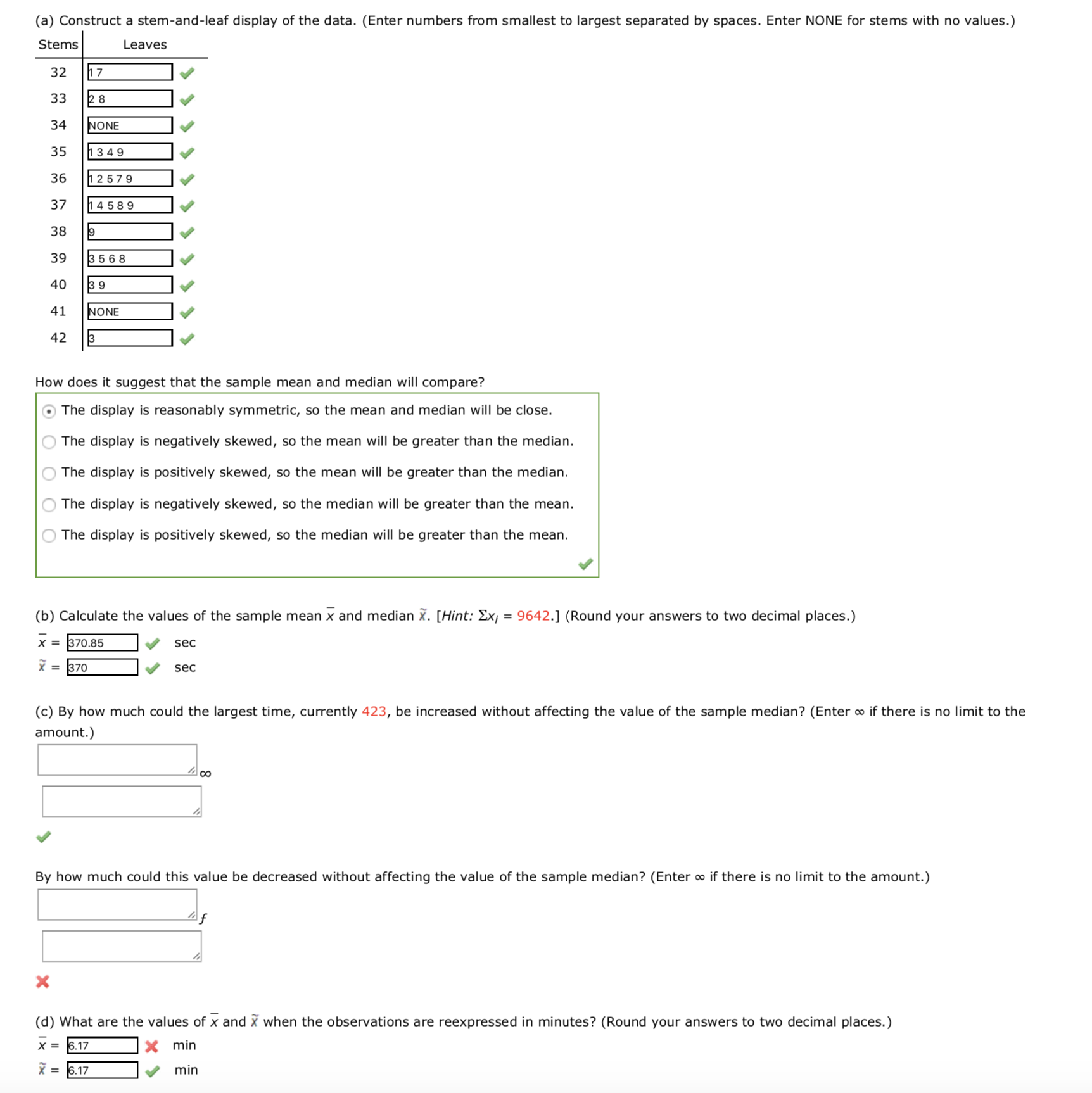# (a) Construct a stem-and-leaf display of the data. (Enter numbers from smallest to largest separated by spaces. Enter NONE for stems with no values.) Stems Leaves 1 . 32 33 CO 34 NONE 35 1349 36 h257 9 37 4589 38 39 3568 40 3 9 NONE 41 42 3 How does it suggest that the sample mean and median will compare? O The display is reasonably symmetric, so the mean and median will be close The display is negatively skewed, so the mean will be greater than the median The display is positively skewed, so the mean will be greater than the median The display is negatively skewed, so the median will be greater than the mean. The display is positively skewed, so the median will be greater than the mean. (b) Calculate the values of the sample mean x and median X. [Hint: £xj = 9642.] (Round your answers to two decimal places.) 370.85 х sec X 370 sec (c) By how much could the largest time, currently 423, be increased without affecting the value of the sample median? (Enter o if there is no limit to the amount.) By how much could this value be decreased without affecting the value of the sample median? (Enter o if there is no limit to the amount.) f X (d) What are the values of x and X when the observations are reexpressed in minutes? (Round your answers to two decimal places.) min = 6.17 х min 6.17

Question

A sample of 26 offshore oil workers took part in a simulated escape exercise, resulting in the accompanying data on time (sec) to complete the escape:

 389 351 354 361 379 423 321 396 403 374 375 371 362 367 365 327 338 395 393 369 378 359 353 409 332 398

I have uploaded a picture with the full problem. I need help in the 2 questions I got wrong. On the picture the table is already set in order from smaller to bigger.

By how much could this value be decreased without affecting the value of the sample median? (Enter ∞ if there is no limit to the amount.) I have no idea on how to calculate that.

The other question is all the way down. I know I have to conver the seconds to minutes. I got 370.85 / 60 = 6.1695 which if I round to 2 decimal places as asked by the problem I should be getting 6.17 minutes. I was marked wrong. Why?

Thanks.help_outlineImage Transcriptionclose(a) Construct a stem-and-leaf display of the data. (Enter numbers from smallest to largest separated by spaces. Enter NONE for stems with no values.) Stems Leaves 1 . 32 33 CO 34 NONE 35 1349 36 h257 9 37 4589 38 39 3568 40 3 9 NONE 41 42 3 How does it suggest that the sample mean and median will compare? O The display is reasonably symmetric, so the mean and median will be close The display is negatively skewed, so the mean will be greater than the median The display is positively skewed, so the mean will be greater than the median The display is negatively skewed, so the median will be greater than the mean. The display is positively skewed, so the median will be greater than the mean. (b) Calculate the values of the sample mean x and median X. [Hint: £xj = 9642.] (Round your answers to two decimal places.) 370.85 х sec X 370 sec (c) By how much could the largest time, currently 423, be increased without affecting the value of the sample median? (Enter o if there is no limit to the amount.) By how much could this value be decreased without affecting the value of the sample median? (Enter o if there is no limit to the amount.) f X (d) What are the values of x and X when the observations are reexpressed in minutes? (Round your answers to two decimal places.) min = 6.17 х min 6.17 fullscreen